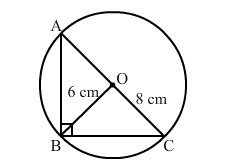# Find the area of a right-angled triangle, the radius of whose circumcircle measure 8 cm`
Question:

Find the area of a right-angled triangle, the radius of whose circumcircle measure 8 cm and the altitude drawn to the hypotenuse measures 6 cm.

Solution:​Height = 6 cm
Area=?
In a right-angled triangle, the centre of the circumcircle is the mid-point of the hypotenuse.

Hypotenuse $=2 \times$ (radius of circumcircle) for a right triangle

$=2 \times 8$

$=16 \mathrm{~cm}$

So, hypotenuse $=16 \mathrm{~cm}$

Now, base = 16 cm and height = 6 cm

Area of the triangle $=\frac{1}{2} \times$ base $\times$ height

$=\frac{1}{2} \times 16 \times 6$

$=48 \mathrm{~cm}^{2}$